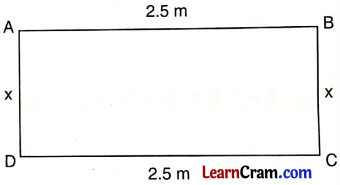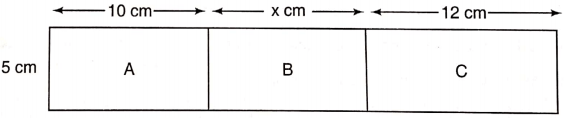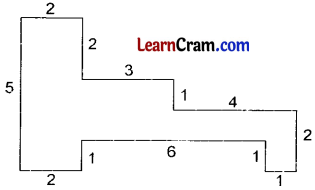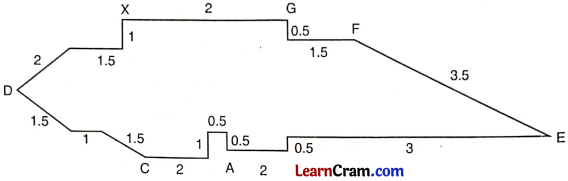# DAV Class 6 Maths Chapter 15 Brain Teasers Solutions

The DAV Class 6 Maths Book Solutions and DAV Class 6 Maths Chapter 15 Brain Teasers Solutions of Perimeter and Area offer comprehensive answers to textbook questions.

## DAV Class 6 Maths Ch 15 Brain Teasers Solutions

Question 1.
A. Tick (✓) the correct answer.
(a) The length of the side of a square whose perimeter is 32 cm is:
(i) 16 cm
(ii) 8 cm
(iii) 48 cm
(iv) 128 cm
(ii) 8 cm.
Perimeter of the square = 32 cm.
Side of the square = $$\frac{\text { Perimeter of square }}{4}$$
= $$\frac{32}{4}$$
= 8 cm

(b) Perimeter of the following rectangle is 800 cm, then the value of x is:(i) 150 cm
(ii) 250 cm
(iii) 25 cm
(iv) 5 cm
(i) 150 cm.

Perimeter of rectangle = 2 (l + b)
⇒ 800 cm = 2(2.5 m + x)
⇒ 800 cm = 2(2.5 x 100 cm + x)
⇒ 800 cm = 2(250 cm + x)
⇒ 250 cm + x = $$\frac{800}{2}$$ = 400 cm
⇒ x = 400 – 250 = 150 cm.

(c) Sum of the areas of regions A, B and C is 140 cm2, then x is:(i) 30 cm
(ii) 6 cm
(iii) 8 cm
(iv) 40 cm
(ii) 6 cm.

Regions A, B and C form a rectangle.
Thus, area of rectangular region
Here, length – 10 cm + x + 12 cm
= 22 cm + x
And breath = 5 cm.
Area of rectangular regions = (22 cm + x) × 5 cm
140cm = (22 cm + x) 5 cm
⇒ 22 cm + x = $$\frac{140 \mathrm{~cm}^2}{5 \mathrm{~cm}}$$ = 28 cm
⇒ x = 28 cm – 22 cm = 6 cm.

(d) If the length of a rectangle is doubled keeping the breath the same, then its area is—
(i) doubled
(ii) unchanged
(iii) halved
(iv) squared
(i) doubled.
Area of rectangle = l × b
on doubling the length keeping the breadth the same.
Area of new rectangle = 2l × b
Thus,
$$\frac{\text { Area of rectangle }}{\text { Area of new rectangle }}=\frac{l \times b}{2 l \times b}=\frac{1}{2}$$
⇒ Area of new rectangle
= 2 × Area of rectangle

(e) The perimeter of the given figure is:(i) 26 units
(ii) 28 units
(iii) 30 units
(iv) 32 units
(iii) 30 unitsPerimeter of the given figure = sum of all lengths
= 5 + 2 + 2 + 3 + 1 + 4 + 2 + 1 + 1 + 6 + 1 + 2
= 30 units.(a) Into how many square of side 2 cm each can you divide a rectangle of length 8 cm and breadth 4 cm?
Length of rectangle = 8 cm
Breadth of rectangle = 4 cm
Area of the rectangle = l × b
= 8 cm × 4 cm
= 32 cm2
Side of the square = 2 cm
Area of the square = (side)2 = (2 cm)2
= 4 cm2
No. ot square = $$\frac{\text { Area of rectangle }}{\text { Area of square }}$$
= $$\frac{32 \mathrm{~cm}^2}{4 \mathrm{~cm}^2}$$
= 8

(b) What is the side of the square whose area is 36 cm2?
Area of the square = 36 cm2
Side of the square = $$\sqrt{\text { Area of the square }}$$
= $$\sqrt{36}=\sqrt{6 \times 6}=\sqrt{6^2}$$
= 6 cm.

(c) A piece of string is 120 cm long. If the string is bent to form a square, what will be the length of each side?
Perimeter of square = 120 cm
Length of each side of square
= $$\frac{\text { Perimeter of the square }}{4}$$
= $$\frac{120 \mathrm{~cm}}{4}$$ = 30 cm

(d) Find the area of the shaded region.Area of the region = side × side
= 10 cm × 10 cm
= 100 cm2

Area of squares of four corners = 4 (side × side)
= 4 × (1 × 1)
= 4 × 1 cm2
= 4 cm2
Thus, area of shaded regions = 100 cm2 – 4 cm2
= 96 cm2.

(e) Find the perimeter of a rectangle of length 1.5 m and breadth 50 cm.
Length of rectangle = 1.5 m
= 1.5 × 100 cm
= 150 cm
Breadth of rectangle = 50 cm
Thus, perimeter of rectangle = 2 (l + b)
= 2(150 + 50)
= 2 × 200 cm
= 400 cm.

Question 2.
Which of the following has larger area and by how much? A rectangle of length 42 cm and breadth 28 cm.
or
A square of side 39 cm.
Area of the rectangle = length × breadth
= 42 cm × 28 cm
= 1176 cm2

Area of the square = (side)2
= (39)2
= 1521 cm2
It is clear that area of the square is greater than the area of the rectangle by 1521 – 1176
= 345 cm2.

Question 3.
What will happen to the area of a square when
(a) its side is doubled?
(b) its side is halved?
Let the side of the square be a cm its area = (side)2 = a2 cm2
(a) If its side is 2a cm
then its area = (2a)2 = 4a2 cm2
∴ its area becomes 4 times.

(b) If its side is $$\frac{a}{2}$$ cm
then its area $$\left(\frac{a}{2}\right)^2=\frac{a^2}{4}$$cm2
∴ its area will become one fourth.Question 4.
The area of a rectangle is 1560 cm2 and its length is 60 cm. Find its breadth.
Area of the rectangle = 1560 cm2
Length of the rectangle = 60 cm
∴ Breadth = $$\frac{\text { Area }}{\text { Length }}$$ = $$\frac{1560}{60}$$ = 26 cm
Hence the required breadth = 26 cm

Question 5.
The area of a square of side 8 cm is the same as that of a rectangle of length 32 cm. What is the breadth of the rectangle?
Area of the square = Area of the rectangle
(8)2 = Area of the rectangle
64 = Area of the rectangle
∴ Breadth of the rectangle = $$\frac{64}{\text { Length }}$$
= $$\frac{64}{32}$$ = 2 cm
Hence the required breadth = 2 cm

Question 6.
A square tile is having side of length 15 cm. How many such tiles would be required to cover the square floor of a bathroom of side 3 m?
Length of side of square tile =15 cm
∴ its area = 15 × 15
= 225 cm2
Area of the floor of bathroom = 3 × 3
= 9 m2
= 9 × 100 × 100 cm2
= 90000 cm2
Number of tiles required
= $$\frac{90000}{225}$$ = 400
Hence the required number of tiles = 400

Question 7.
Find the area of a square lawn whose perimeter is 48 m.
Perimeter of the square lawn = 48 m
∴ Side of the lawn = $$\frac{48}{4}$$m = 12 m
∴ Area of the square lawn = (Side)2 = (12)2 = 144 m2
Hence the required area = 144 m2

Question 8.
Fire broke out at point A. Fire extinguisher is at point X. Which route (in km) should take to reach point A?Distance of XDCA = 1 + 1.5 + 2 + 1.5 + 1 + 1.5 + 2 + 1 + 0.5 + 0.5
= 12.5 km
Distance of XGFEA = 2 + 0.5 + 1.5 + 3.5 + 3 + 0.5 + 2
= 13 km
Distance of XGFEA > Distance of XDCA.
So, fire extinguisher should take route XDCA

### DAV Class 6 Maths Chapter 1 HOTS

Question 1.
A street lane is to be paved with tiles of length 12 cm and breadth 10 cm. If the length of the lane is 240 m and its breadth is 12 m, find the number of tiles needed.
Area of tiles = l × b
= 12 cm × 10 cm
= 120 cm2

Length (L) of the lane = 240 m
= 240 × 100 cm
= 24000 cm

Breadth (B) of the lane = 12 m
= 12 × 100 cm
= 1200 cm

Area of the lane = L × B
= 24000 cm × 1200 cm
= 28800000 cm2

Thus, the number of tiles should be needed to pave the lane
= $$\frac{\text { Area of the lane }}{\text { Area of a tile }}=\frac{28800000 \mathrm{~cm}^2}{120 \mathrm{~cm}^2}$$
= 2,40,000 tilesQuestion 2.
A wire of length 20 cm is to be bent to form a rectangle of
(i) maximum area
(ii) minimum area If the length and breadth are taken always in natural numbers, find the same in each case.
Sol. Perimeter of rectangle = 20 cm
2(l + b) = 20 ⇒ l + b = = 10 cm
On putting b = 1 cm, 2 cm, 3 cm, 4 cm, 5 cm, …. respectively we get l = 9 cm, 8 cm, 7 cm, 6 cm, 5 cm, …. respectively
(i) Maximum area when l and b are equals, i.e. 1 = 5 cm, b = 5 cm
Maximum area = 5 × 5 = 25 cm2
(ii) Minimum area when 1 = 9 cm and b = 1 cm
∴ Minimum area = l × b = 9 × 1 = 9 cm2

Question 1.
The length and breadth of a rectangular sheet are 24 cm and 16 cm respectively.
(i) Find the area of the sheet.
Length of the sheet = 24 cm
Breadth of the sheet = 16 cm
Area of the sheet = l × b
= 24 cm × 16 cm
= 384 cm2

(ii) If the sheet is made a square with side 16 cm, what will be the area of the square sheet?
Each side of the square sheet =16 cm its area = (side)2
= (16)2
= 256 cm2

(iii) What will be the area of the separated sheet?
Length of the separated sheet = 16 cm and the breadth = 24 cm – 16 cm = 8 cm
Area of this sheet = l × b
= 16 cm × 8 cm
= 128 cm2

(iv) What will be the ratio of the areas of the two sheets?
Area of the square sheet = 256 cm2
Area of the rectangular sheet = 128 cm2
Ratio between two area = 256 cm2 : 128 cm2 = 2:1

Question 2.
The perimeter of a square is 64 cm. Find its area.
Perimeter of a square = 64 cm
its side = $$\frac{\text { Perimeter }}{4}=\frac{64}{4}$$ = 16 cm
Area of this square = (Side)2 = (16)2
= 256 cm2
Hence the required area = 256 cm2

Question 3.
The area of a rectangular field is 324 m2. If its length is 27 m, find its (i) Breadth (ii) Perimeter.
Area of the rectangular field = 324 m2
Length of the field = 27 m
(i) ∴ Breadth of the field = $$\frac{\text { Area }}{\text { Length }}=\frac{324}{27}$$
= 12 m
(ii) Perimeter of the rectangular field = 2 [l + b]
= 2[27 + 12] m
= 2 × 39 m
= 78 mQuestion 4.
The length and breadth of a rectangular field are 22 m and 14 m respectively. Find the cost of fencing it at a rate of ? 15 per metre.
Length of the field = 22 m
Breadth of the field = 14 m
Perimeter of the field = 2[l + b]
= 2 [22 + 14]
= 2 × 36
= 72 m
Cost of fencing the field = ₹ (15 × 72) = ₹ 1080
Hence the required cost = ₹ 1080

Question 5.
The cost of fencing of a rectangular field at the rate of ₹ 20 per metre is ₹ 1080. Find the perimeter of the field.
₹ 1080 will be the cost of $$\frac{1080}{20}$$ meter of fencing = 54 m
ite Side = $$\frac{\text { Perimeter }}{4}=\frac{96}{4}$$ m = 24 m